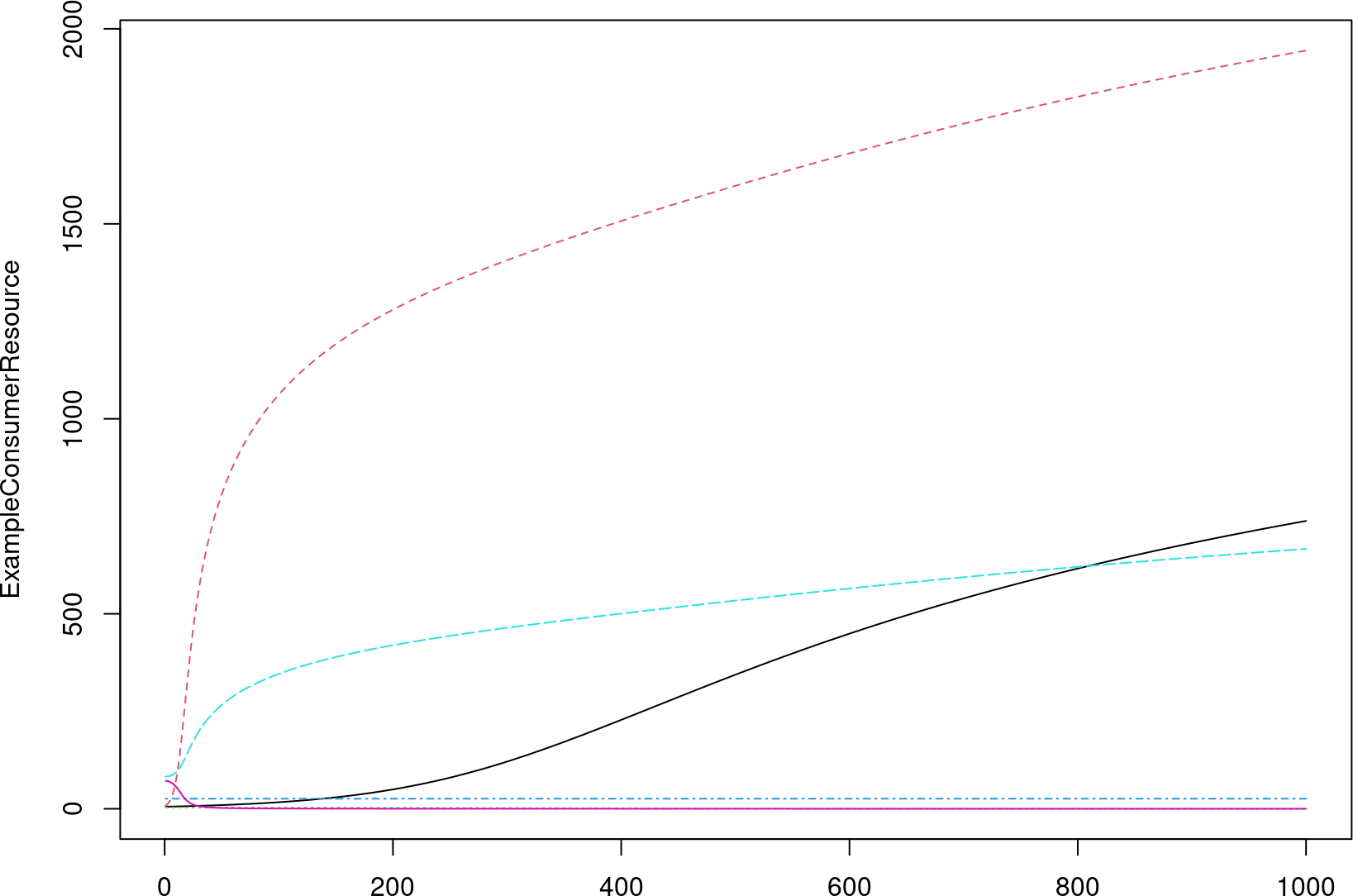# 1 Introduction

miaSim implements tools for microbiome data simulation based on the microsim .

Microbiome time series simulation can be obtained by generalized Lotka-Volterra model,simulateGLV, and Self-Organized Instability (SOI), simulateSOI. Hubbell’s Neutral model, simulateHubbell is used to determine the species abundance matrix.

The resulting abundance matrix from these three simulation models can be implemented to SummarizedExperiment object or TreeSummarizedExperiment object by using convertToSE.

powerlawA and randomA give interaction matrix of species generated by normal distribution and uniform distribution, respectively. These matrices can be used in the simulation model examples.

simulationTimes generates lists of time series that can be specified as simulation time and time points to keep in simulated time.

# 2 Installation

### 2.0.1 Bioc-release

if (!requireNamespace("BiocManager", quietly = TRUE))
install.packages("BiocManager")

# 3 Models for simulating microbiome data sets

simulateGLV is the generalized Lotka-Volterra simulation model fitted to time-series estimates microbial population dynamics and relative rates of interaction. The model relies on interaction matrix that represents interaction heterogeneity between species. This interaction matrix can be generated with powerlawA or randomA functions depending on the distribution method.

The function powerlawA uses normal distribution to create interaction matrix.

library(miaSim)
A_normal <- powerlawA(n_species = 12, alpha = 3)

The function randomA uses uniform distribution to create interaction matrix.

A_uniform <- randomA(n_species = 10, d = -0.4, min_strength = -0.8,
max_strength = 0.8, connectance = 0.5)

The number of species specified in the interaction matrix must be the same amount as the species used in the simulateGLV and simulateSOI models. simulateGLV and simulateRicker functions both use the generalized Lotka-Volterra model.

ExampleGLV <- simulateGLV(n_species = 12, A_normal, t_start = 0,
t_store = 1000, stochastic = FALSE, norm = FALSE)

ExampleRicker <- simulateRicker(n_species=12, A_normal, tend=100, norm = FALSE)

Time series is added to simulateGLV with simulationTimes function where the time points can be kept and extracted from simulation time as a separate list.

Time <- simulationTimes(t_start = 0, t_end = 100, t_step = 0.5,
t_store = 100)
Time$t.index  ## NULL simulateHubbell includes the Hubbell Neutral simulation model which explains the diversity and relative abundance of species in ecological communities. This model is based on the community dynamics; migration, births and deaths. Loss in society is either replaced by migration or birth. Similarly, simulateHubbellRates also uses the Hubbell Neutral model.Migration is determined by the metacommunity and replacement by birth is measure by growth rates. The time between events is the determinant of replacement. ExampleHubbell <- simulateHubbell(n_species = 8, M = 10, I = 1000, d = 50, m = 0.02, tend = 100) ExampleHubbellRates <- simulateHubbellRates(community_initial = c(0,5,10), migration_p = 0.1, metacommunity_p = NULL, k_events = 1, growth_rates = NULL, norm = FALSE, t_end=1000) The Self-Organised Instability (SOI) model can be found in simulateSOI and it generates time series for communities and accelerates stochastic simulation. ExampleSOI <- simulateSOI(n_species = 12, I = 1000, A_normal, k=5, com = NULL, tend = 150, norm = TRUE) Stochastic logistic model is used in simulateStochasticLogistic to determine dead and alive counts in community. ExampleLogistic <- simulateStochasticLogistic(n_species = 5) The consumer resource community simulation model simulateConsumerResource requires the use of the randomE function, which returns a matrix containing the production rates and consumption rates of each species. The resulting matrix is used as a determination of resource consumption efficiency. ExampleConsumerResource <- simulateConsumerResource(n_species = 2, n_resources = 4, eff = randomE(n_species = 2, n_resources = 4)) # visualize the dynamics of the model Consumer_plot <- matplot(ExampleConsumerResource, type = "l")The community simulations result in abundance matrix that can be stored in SummarizedExperiment [@SE] class object. Other fields, such as rowData containing information about the samples, and colData, consisting of sample metadata describing the samples, can be added to the SummarizedExperiment class object. This can be done by conversion function convertToSE. ExampleHubbellRates <- simulateHubbellRates(community_initial = c(0,5,10), migration_p = 0.1, metacommunity_p = NULL, k_events = 1, growth_rates = NULL, norm = FALSE, t_end=1000) HubbellSE <- convertToSE(assay = ExampleHubbellRates$counts,
colData = ExampleHubbellRates$time, metadata = ExampleHubbellRates$metadata)

Also, the TreeSummarizedExperiment class object can be created using the required hierarchical information in addition to the SummarizedExperiment class object. It can also be reached through convertToSE.

For further details:

After converting to SummarizedExperiment or
TreeSummarizedExperiment the models can be used with: various visualization functions under miaViz, many analysis tools that are available under mia and time series analysis tools under miaTime.

miaViz installation

mia installation

HubbellSE population density can be plotted with plotAbundanceDensity function from miaViz.

ExampleGLV <- simulateGLV(n_species = 12, A_normal, t_start = 0,
t_store = 1000, stochastic = FALSE, norm = FALSE)

rownames(ExampleGLV) <- c(paste("Species", rownames(ExampleGLV), sep = "_"))
colnames(ExampleGLV) <- c(paste("Sample", seq_len(ncol(ExampleGLV)), sep = "_"))

df <- DataFrame(sampleID = colnames(ExampleGLV),
Time = seq(1, 1000, 1),
SubjectID = rep(1:4, 250),
row.names = colnames(ExampleGLV))

SE_GLV <- convertToSE(assay = ExampleGLV,
colData = df)

More examples on SummarizedExperiment object manipulation and analysis can be found at https://microbiome.github.io/OMA/ .

# 4 Session info

sessionInfo()
## R version 4.2.1 (2022-06-23)
## Platform: x86_64-pc-linux-gnu (64-bit)
## Running under: Ubuntu 20.04.5 LTS
##
## Matrix products: default
## BLAS:   /home/biocbuild/bbs-3.16-bioc/R/lib/libRblas.so
## LAPACK: /home/biocbuild/bbs-3.16-bioc/R/lib/libRlapack.so
##
## locale:
##   LC_CTYPE=en_US.UTF-8       LC_NUMERIC=C
##   LC_TIME=en_GB              LC_COLLATE=C
##   LC_MONETARY=en_US.UTF-8    LC_MESSAGES=en_US.UTF-8
##   LC_PAPER=en_US.UTF-8       LC_NAME=C
##  LC_MEASUREMENT=en_US.UTF-8 LC_IDENTIFICATION=C
##
## attached base packages:
##  stats4    stats     graphics  grDevices utils     datasets  methods
##  base
##
## other attached packages:
##   miaSim_1.4.0                   TreeSummarizedExperiment_2.6.0
##   Biostrings_2.66.0              XVector_0.38.0
##   SingleCellExperiment_1.20.0    SummarizedExperiment_1.28.0
##   Biobase_2.58.0                 GenomicRanges_1.50.0
##   GenomeInfoDb_1.34.0            IRanges_2.32.0
##  S4Vectors_0.36.0               BiocGenerics_0.44.0
##  MatrixGenerics_1.10.0          matrixStats_0.62.0
##  BiocStyle_2.26.0
##
## loaded via a namespace (and not attached):
##   Rcpp_1.0.9             ape_5.6-2              lattice_0.20-45
##   tidyr_1.2.1            gtools_3.9.3           assertthat_0.2.1
##   digest_0.6.30          utf8_1.2.2             R6_2.5.1
##  pracma_2.4.2           evaluate_0.17          highr_0.9
##  pillar_1.8.1           yulab.utils_0.0.5      zlibbioc_1.44.0
##  rlang_1.0.6            lazyeval_0.2.2         jquerylib_0.1.4
##  magick_2.7.3           Matrix_1.5-1           rmarkdown_2.17
##  BiocParallel_1.32.0    stringr_1.4.1          RCurl_1.98-1.9
##  DelayedArray_0.24.0    compiler_4.2.1         xfun_0.34
##  pkgconfig_2.0.3        htmltools_0.5.3        tidyselect_1.2.0
##  tibble_3.1.8           GenomeInfoDbData_1.2.9 bookdown_0.29
##  codetools_0.2-18       fansi_1.0.3            crayon_1.5.2
##  dplyr_1.0.10           bitops_1.0-7           grid_4.2.1
##  nlme_3.1-160           jsonlite_1.8.3         lifecycle_1.0.3
##  DBI_1.1.3              magrittr_2.0.3         tidytree_0.4.1
##  cli_3.4.1              stringi_1.7.8          cachem_1.0.6
##  bslib_0.4.0            generics_0.1.3         vctrs_0.5.0
##  deSolve_1.34           tools_4.2.1            treeio_1.22.0
##  glue_1.6.2             purrr_0.3.5            poweRlaw_0.70.6
##  parallel_4.2.1         fastmap_1.1.0          yaml_2.3.6
##  BiocManager_1.30.19    knitr_1.40             sass_0.4.2